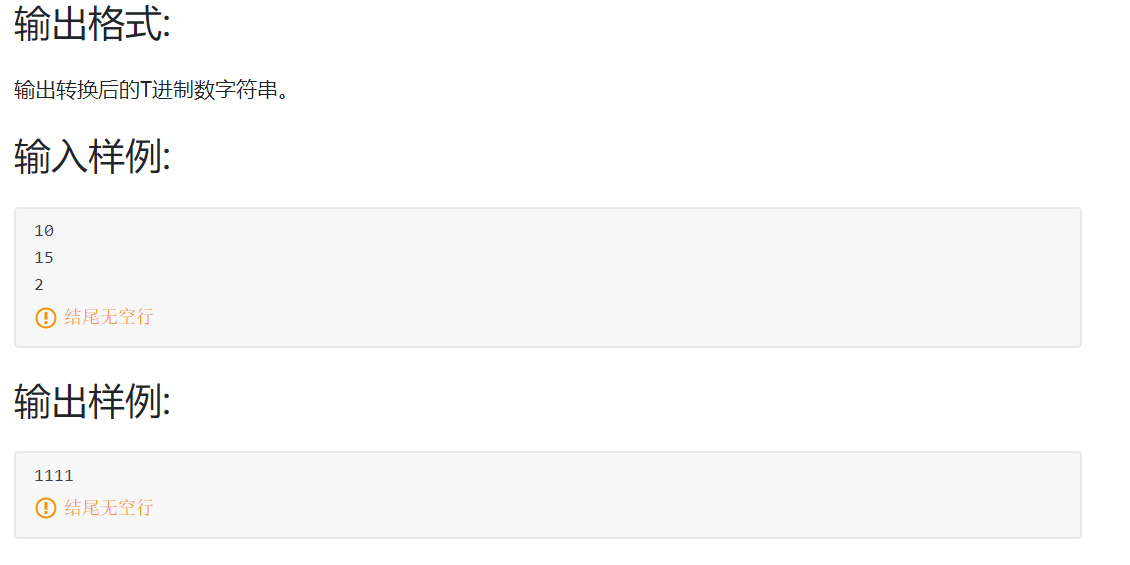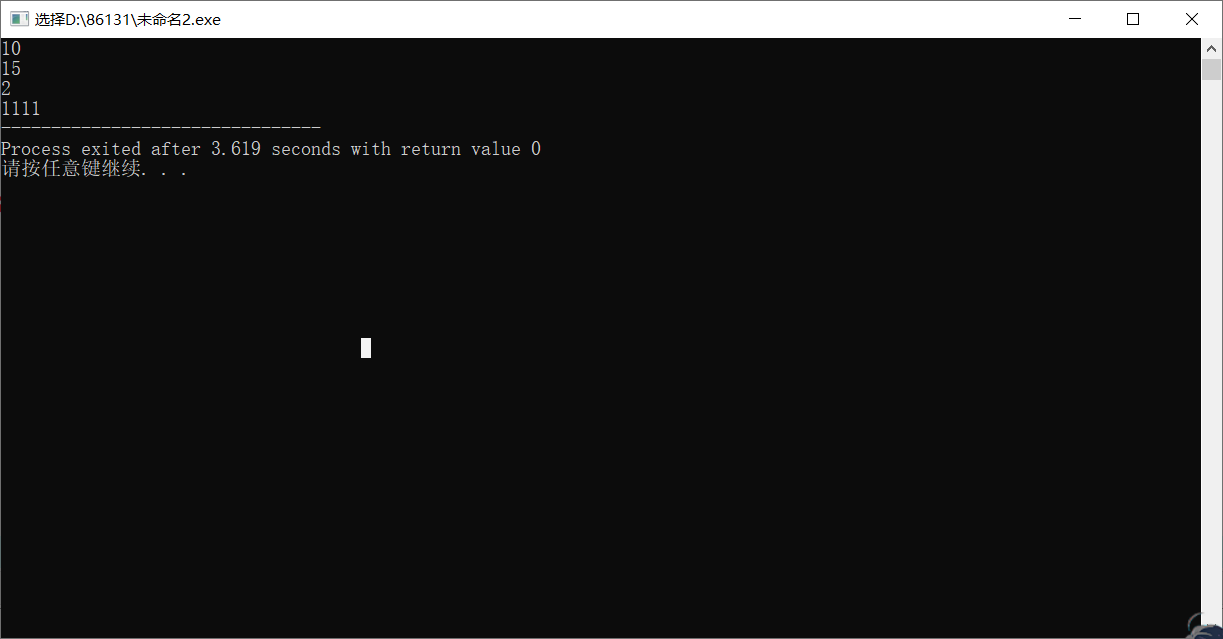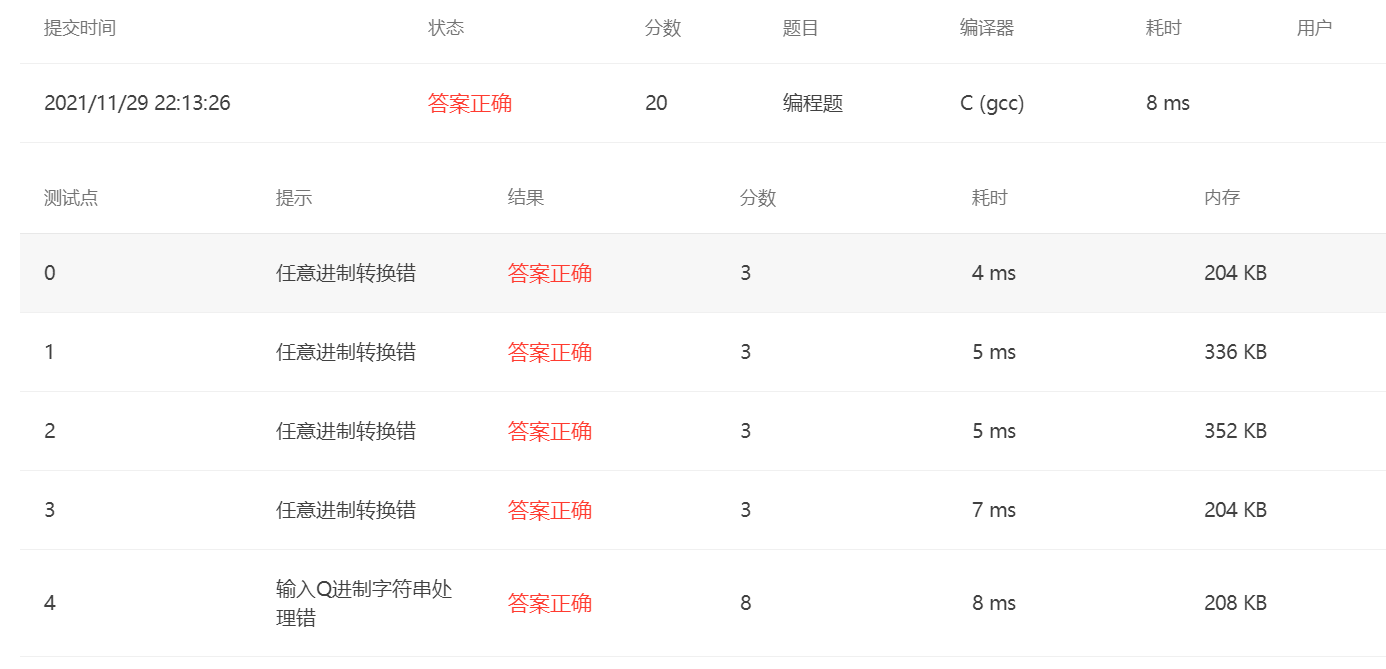# 进制转换（Q进制转换成T进制）

### 输入格式:#include <stdio.h>
int main() {
int Q, T, sum = 0;
scanf("%d", &Q);
getchar();
char ch;
while ((ch = getchar()) != '\n')
if (ch >= '0' && ch <= ('0' + Q - 1))
sum = sum * Q + (ch - '0');
if (sum == 0) {
printf("0\n");
return 0;
}
scanf("%d", &T);
if (T == 10) {
printf("%d\n", sum);
return 0;
}
int cur = 0, a;
while (sum != 0) {
a[cur++] = sum % T;
sum /= T;
}
for (int i = cur - 1; i >= 0; i--)
printf("%d", a[i]);
return 0;
}posted @ 2021-11-29 22:22  查喔1959  阅读(54)  评论(0编辑  收藏  举报